## 14.5.1 Problem Definition

Formulation 14.1 assumed that feedback is not necessary. If the initial state is given, then the solution takes the form of an action trajectory, which upon integration yields a time-parametrized path through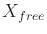. This extended the Piano Mover's Problem of Section 4.3.1 to include phase spaces and differential constraints. Now suppose that feedback is required. The reasons may be that the initial state is not given or the plan execution might not be predictable due to disturbances or errors in the system model. Recall the motivation from Section 8.1.

With little effort, the feedback motion planning framework from Chapter 8 can be extended to handle differential constraints. Compare Formulations 8.2 and 14.1. Feedback motion planning under differential constraints is obtained by making the following adjustments to Formulation 8.2:

1. In Formulation 8.2,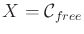, which automatically removed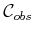fromby definition. Now letbe any C-space or phase space, and let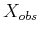be defined as in Formulation 8.2. This leads to, as defined in Formulation 14.1.
2. In Formulation 8.2, the state transition equation was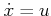, which directly specified velocities in the tangent space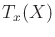. Now let any system,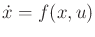, be used instead. In this case,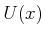is no longer a subset of. It still includes the special termination action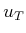.
3. Formulation 14.1 includes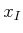, which is now removed for the feedback case to be consistent with Formulation 8.2.
4. A feedback plan is now defined as a function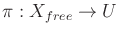. For a given state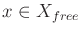, an action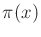is produced. Composing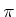with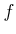yields a velocity ingiven by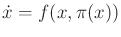. Therefore,defines a vector field on.
Let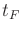denote the time at whichis applied. Both feasible and optimal planning can be defined using a cost functional,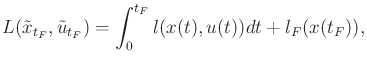(14.26)

which is identical to that given in Section 8.4.1. This now specifies the problem of feedback motion planning under differential constraints.

The most important difference with respect to Chapter 8 is thatis replaced with, which allows complicated differential models of Chapter 13 to be used. The vector field that results frommust satisfy the differential constraints imposed by. In Section 8.4.4, simple constraints on the allowable vector fields were imposed, such as velocity bounds or smoothness; however, these constraints were not as severe as the models in Chapter 13. For example, the Dubins car does not allow motions in the reverse direction, whereas the constraints in Section 8.4.4 permit motions in any direction.

Steven M LaValle 2020-08-14